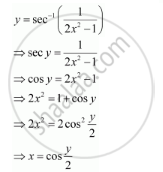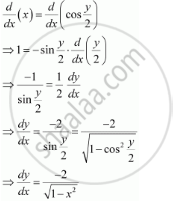Share

# Find Dy/Dx for Y = Sec^(-1) (1/(2x^2 - 1)), 0 < X < 1/Sqrt2 - CBSE (Science) Class 12 - Mathematics

ConceptDerivatives of Inverse Trigonometric Functions

#### Question

Find dy/dx

y = sec^(-1) (1/(2x^2 - 1)), 0 < x < 1/sqrt2

#### Solution

The given relationship is y = sec^(-1) (1/(2x^2 - 1))Differentiating this relationship with respect to x, we obtainIs there an error in this question or solution?

#### APPEARS IN

NCERT Solution for Mathematics Textbook for Class 12 (2018 to Current)
Chapter 5: Continuity and Differentiability
Q: 15 | Page no. 169

#### Video TutorialsVIEW ALL 

Solution Find Dy/Dx for Y = Sec^(-1) (1/(2x^2 - 1)), 0 < X < 1/Sqrt2 Concept: Derivatives of Inverse Trigonometric Functions.
S